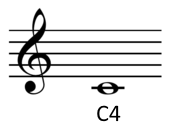{{announcement.body}}
{{announcement.title}}

# Converting Sound Frequency to Musical Pitch Notation

DZone 's Guide to

# Converting Sound Frequency to Musical Pitch Notation

### Using Python to have code output musical pitch notation based on sound frequencies.

· Big Data Zone ·
Free Resource

Comment (0)

Save
{{ articles.views | formatCount}} Views

How can you convert the frequency of a sound to musical notation? I wrote in an earlier post how to calculate how many half steps a frequency is above or below middle C, but it would be useful go further have code to output musical pitch notation.

In scientific pitch notation, the C near the threshold of hearing, around 16 Hz, is called C0. The C an octave higher is C1, the next C2, etc. Octaves begin with C; other notes use the octave number of the closest C below.The lowest note on a piano is A0, a major sixth up from C0. Middle C is C4 because it’s 4 octaves above C0. The highest note on a piano is C8.

## Math

A4, the A above middle C, has a frequency of 440 Hz. This is nine half steps above C4, so the pitch of C4 is 440*2-9/12. C0 is four octaves lower, so it’s 2-4 = 1/16 of the pitch of C4. (Details for this calculation and the one below are given in here.)

For a pitch P, the number of half steps from C0 to P is h = 12 log2(P / C0).

## Software

The Python code below calculates the number of half steps h from C0 up to a pitch, then computes the corresponding pitch notation.

``````from math import log2, pow

A4 = 440
C0 = A4*pow(2, -4.75)
name = ["C", "C#", "D", "D#", "E", "F", "F#", "G", "G#", "A", "A#", "B"]

def pitch(freq):
h = round(12*log2(freq/C0))
octave = h // 12
n = h % 12
return name[n] + str(octave)
``````

The pitch for A4 is its own variable in case you’d like to modify the code for a different tuning. While 440 is common, it used to be lower in the past, and you’ll sometimes see higher values like 444 today.

If you’d like to port this code to a language that doesn’t have a `log2` function, you can use `log(x)/log(2)` for `log2(x)`.

## Powers of 2

When scientific pitch notation was first introduced, C0 was defined to be exactly 16 Hz, whereas now it works out to around 16.35. The advantage of the original system is that all C’s have frequency a power of 2, i.e. Cn has frequency 2n+4 Hz. The formula above for the number of half steps a pitch is above C0 simplifies to h = 12 log2P – 48.

If C0 has frequency 16 Hz, the A above middle C has frequency 28.75 = 430.54, a little flat compared to A 440. But using the A 440 standard, C0 = 16 Hz is a convenient and fairly accurate approximation.

Topics:
simulation ,statistics ,contest

Comment (0)

Save
{{ articles.views | formatCount}} Views

Published at DZone with permission of

Opinions expressed by DZone contributors are their own.